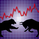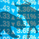จำนวนเข้าชม 2562
2562
Revision: 2

Description:
• Designed for quick and clear visual analysis of market direction, aiming to highlight areas suitable for entry/exit.
• Channel width's are based on the average high and low difference over X period, and as such can help determine reasonable target price and stop distances.
• There is no specific strategy to use with this indicator, it is only intended to help bolster confidence in your existing methods, especially for trend traders and scalpers.

Usage/setup:
• Option to use alternate time-frame(s).
• Multitude of MA types available for the basis, and relevant inputs.
• High-Low difference period - longer is smoother, but shorter will more accurately gauge current market variance.
• Colour swap delay period - Basis MA value must change up/down beyond average for X period in order for change to occur.
• % high-low channel values - a value of 1.0 is equal to 100% of the average high-low difference for selected basis period.
• "Transient" channel colouration helps pick out lows in up-trends and highs in down-trends for suitable entry locations. This should be set to a lower resolution than the main channel.
```//@version=2

study(title="High Low Difference Trend Assist V2", shorttitle="HLD Trend Assist", overlay=true)

// Revision:    2
// Author:      JayRogers
//
// Description:
//  - Designed for quick and clear visual analysis of market direction, aiming to highlight areas suitable for entry/exit
//  - Channel width's are based on the average high and low difference over X period, and as such can help determine
//    reasonable target price and stop distances.
//  - There is no specific strategy to use with this indicator, it is only intended to help bolster confidence in your
//    existing methods, especially for trend traders and scalpers.
// Usage/setup:
//  - Option to use alternate time-frame(s).
//  - Multitude of MA types available for the basis, and relevant inputs.
//  - High-Low difference period - longer is smoother, but shorter will more accurately guage current market variance.
//  - Colour swap delay period - Basis MA value must change up/down beyond average for X period in order for change to occur.
//  - % high-low channel values - a value of 1.0 is equal to 100% of the average high-low difference for selected basis period.
//  - "Transient" channel colouration helps pick out lows in uptrends and highs in downtrends for suitable entry locations.
//    This should be set to a lower resolution than the main channel

// === INPUTS ===
//useRes1     = input(defval = true, title = " Resolution?")
mainRes     = input(defval = "60", title = "Main Channel Resolution", type = resolution)
transRes    = input(defval = "30", title = "Transient Channel Resolution", type = resolution)
basisType   = input(defval = "TEMA", title = "Basis Type: SMA, EMA, DEMA, TEMA, WMA, VWMA, SMMA, HullMA, LSMA, ALMA ( case sensitive )", type = string)
basisSrc    = input(defval = open, title = "Basis Source", type=source)
basisLen    = input(defval = 64, title = "Basis Period", minval = 1)
smoothLen   = input(defval = 200, title = "High-Low Average Difference Period ( higher is smoother )", minval = 1)
offsetSigma = input(defval = 6, title = "Offset for LSMA / Sigma for ALMA", minval = 0)
offsetALMA  = input(defval = 0.85, title = "Offset for ALMA", minval = 0, step = 0.01)
colSwitch   = input(defval = 7, title = "Basis MA Switch Period ( direction confirmation )", minval = 0)
multInner   = input(defval = 1.0, title = "Center Channel Width ( % high-low )", minval = 0.05, step = 0.05)
multOffset  = input(defval = 3.0, title = "Outer Channels Offset ( % high-low )", minval = 1, step = 0.05)
multOuter   = input(defval = 1.0, title = "Outer Channels Width ( % high-low )", minval = 0.1, step = 0.05)
multTrans   = input(defval = 1.0, title = "Transient Channel Width ( % high-low )", minval = 0.1, step = 0.05)
// === /INPUTS ===

// === BASE FUNCTIONS ===
// Returns MA input selection variant, default to SMA if blank or typo.
variant(type, src, len, offSig, offALMA) =>
v1 = sma(src, len)                                                  // Simple
v2 = ema(src, len)                                                  // Exponential
v3 = 2 * v2 - ema(v2, len)                                          // Double Exponential
v4 = 3 * (v2 - ema(v2, len)) + ema(ema(v2, len), len)               // Triple Exponential
v5 = wma(src, len)                                                  // Weighted
v6 = vwma(src, len)                                                 // Volume Weighted
v7 = na(v5) ? sma(src, len) : (v5 * (len - 1) + src) / len    // Smoothed
v8 = wma(2 * wma(src, len / 2) - wma(src, len), round(sqrt(len)))   // Hull
v9 = linreg(src, len, offSig)                                       // Least Squares
v10 = alma(src, len, offALMA, offSig)                               // Arnaud Legoux
type=="EMA"?v2 : type=="DEMA"?v3 : type=="TEMA"?v4 : type=="WMA"?v5 : type=="VWMA"?v6 : type=="SMMA"?v7 : type=="HullMA"?v8 : type=="LSMA"?v9 : type=="ALMA"?v10 : v1
// security wrapper for repeat calls
reso(exp, use, res) => use ? security(tickerid, res, exp) : exp
// lagging average switch for MA direction
avgDir(exp, len) =>
average = len >= 2 ? sum(exp, len) / len : exp
up      = exp > average
down    = exp < average
state   = up ? true : down ? false : up
// baseline difference multiplier for offsets
diffMult(base, dif, mult) => base + (dif * mult)
// === /BASE FUNCTIONS ===

// === MAJOR CHANNEL SERIES ===
// base setup
mainBasis   = reso(variant(basisType, basisSrc, basisLen, offsetSigma, offsetALMA), true, mainRes)
basisSwitch = reso(avgDir(mainBasis, colSwitch), true, mainRes)
highLine    = reso(variant(basisType, high, smoothLen, offsetSigma, offsetALMA), true, mainRes)
lowLine     = reso(variant(basisType, low, smoothLen, offsetSigma, offsetALMA), true, mainRes)
difference  = highLine - lowLine
// center channel bands
midUpper    = diffMult(mainBasis, difference, multInner)
midLower    = diffMult(mainBasis, difference, -multInner)
// upper and lower bands
upperInner  = diffMult(mainBasis, difference, multOffset)
upperOuter  = diffMult(mainBasis, difference, (multOffset + multOuter))
lowerInner  = diffMult(mainBasis, difference, -multOffset)
lowerOuter  = diffMult(mainBasis, difference, -(multOffset + multOuter))
// === /MAJOR SERIES ===

// === TRANSIENT CHANNEL SERIES ===
// base setup
transBasis  = reso(variant(basisType, basisSrc, basisLen, offsetSigma, offsetALMA), true, transRes)
transHigh   = reso(variant(basisType, high, smoothLen, offsetSigma, offsetALMA), true, transRes)
transLow    = reso(variant(basisType, low, smoothLen, offsetSigma, offsetALMA), true, transRes)
transDiff   = transHigh - transLow
// center channel bands
transUpper  = diffMult(transBasis, transDiff, multTrans)
transLower  = diffMult(transBasis, transDiff, -multTrans)
// === /TRANSIENT SERIES ===

// === STATE and ZONE LOGIC ===
// overall trend state and switch points
trendState1  = open > midUpper ? true : open < midLower ? false : trendState1
trendState2  = open > transUpper ? true : open < transLower ? false : trendState2
// colour states
trendUpCol      = (trendState1 and trendState2) ? #009900 : (trendState1 and not trendState2) ? #009999 : na
trendDownCol    = (not trendState1 and not trendState2) ? #CC0000 : (not trendState1 and trendState2) ? #CCCC00 : na
// === /STATE and ZONE LOGIC ===

// === PLOTTING ===
barcolor(color = trendUpCol, title = "Trend Up Colours")
barcolor(color = trendDownCol, title = "Trend Down Colours")
// transient channel
plot(transUpper, title = "Transient Channel Upper", color = silver, linewidth = 1, style = circles, transp = 50)
plot(transLower, title = "Transient Channel Lower", color = silver, linewidth = 1, style = circles, transp = 50)
// main basis line
p_mid   = plot(mainBasis, title = "Basis Line", color = basisSwitch ? #009900 : #CC0000, linewidth = 3, style = line, transp = 20)
// central channel in up-trend
p_cu_u  = plot(trendState1 ? midUpper : na, title = "Central Channel Upper (in ut)", color = silver, transp = 100)
p_cl_u  = plot(trendState1 ? midLower : na, title = "Central Channel Lower (in ut)", color = silver, transp = 100)
fill(p_cu_u, p_mid, title = "Central Channel Upper Fill (in ut)", color = silver, transp = 80)
fill(p_cl_u, p_mid, title = "Central Channel Lower Fill (in ut)", color = #009900, transp = 50)
// central channel in down-trend
p_cu_d  = plot(trendState1 ? na : midUpper, title = "Central Channel Upper (in dt)", color = silver, transp = 100)
p_cl_d  = plot(trendState1 ? na : midLower, title = "Central Channel Lower (in dt)", color = silver, transp = 100)
fill(p_cu_d, p_mid, title = "Central Channel Upper Fill (in dt)", color = #CC0000, transp = 50)
fill(p_cl_d, p_mid, title = "Central Channel Lower Fill (in dt)", color = silver, transp = 80)
// upper offset band
p_ub    = plot(upperInner, title = "Outer Upper Band", color = #CC0000, linewidth = 2, style = line, transp = 40)
p_ubx   = plot(upperOuter, transp = 100, editable = false)
fill(p_ub, p_ubx, title = "Upper Channel Fill", color = silver, transp = 80)
// lower offset band
p_lb    = plot(lowerInner, title = "Outer Lower Band", color = #009900, linewidth = 2, style = line, transp = 40)
p_lbx   = plot(lowerOuter, transp = 100, editable = false)
fill(p_lb, p_lbx, title = "Lower Channel Fill", color = silver, transp = 80)
// === /PLOTTING ===```This is awesome, thank you very much for sharing
ตอบกลับI really appreciate your good work ! your scripts are awesome ! it works for stocks as well though - these are meant for currency.. keep on posting more scripts and excellent strategies. I am a big fan of your scripts :-)
ตอบกลับ